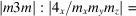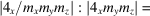International
Tables for
Crystallography
Volume D
Physical properties of crystals
Edited by A. Authier

International Tables for Crystallography (2013). Vol. D, ch. 3.4, p. 492

Section 3.4.2.2.2. Ferroelectric domain states

V. Janoveca* and J. Přívratskáb

aInstitute of Physics, Academy of Sciences of the Czech Republic, Na Slovance 2, CZ-18221 Prague 8, Czech Republic, and bDepartment of Mathematics and Didactics of Mathematics, Technical University of Liberec, Hálkova 6, 461 17 Liberec 1, Czech Republic
Correspondence e-mail:  janovec@fzu.cz

3.4.2.2.2. Ferroelectric domain states

| top | pdf |

Ferroelectric domain states are defined as states with a homogeneous spontaneous polarization; different ferroelectric domain states differ in the direction of the spontaneous polarization. Ferroelectric domain states are specified by the stabilizerof the spontaneous polarizationin the first principal domain state[see equation (3.4.2.16)]:The stabilizeris one of ten polar groups: 1, 2, 3, 4, 6, m,,,,. Sincemust be a polar group too, it is simple to find the stabilizerfulfilling relation (3.4.2.31).

The numberof ferroelectric domain states is given byIf the polar groupdoes not exist, we put. The numberof ferroelectric domain states is given for all ferroic phase transitions in the eighth column of Table 3.4.2.7.

The numberof principal domain states compatible with one ferroelectric domain state (degeneracy of ferroelectric domain states) is given byThe product ofandis equal to the number n of all principal domain states [see equation (3.4.2.19)],The degeneracyof ferroelectric domain states can be calculated for all ferroic phase transitions from the ratio of the numbers n andthat are given in Table 3.4.2.7.

Aizu (1969, 1970a) recognizes three possible cases (see also Table 3.4.2.3):

 (i) Full ferroelectrics: All principal domain states differ in spontaneous polarization. In this case,, i.e., ferroelectric domain states are identical with principal domain states. (ii) Partial ferroelectrics: Some but not all principal domain states differ in spontaneous polarization. A necessary and sufficient condition is, or equivalently,. Ferroelectric domain states are degenerate secondary domain states with degeneracy. In this case, the phase transitioncan be classified as an improper ferroelectric one (see Section 3.1.3.2). (iii) Non-ferroelectrics: No principal domain states differ in spontaneous polarization. There are two possible cases: (a) The parent phase is polar; thenand. (b) The parent phase is non-polar; in this case a polar stabilizerdoes not exist, then we put.

The classification of full-, partial- and non-ferroelectrics and ferroelastics is summarized in Table 3.4.2.3.

Results for all symmetry descents follow readily from the numbers n,,in Table 3.4.2.7and are given for all symmetry descents in Aizu (1970a). One can conclude that partial ferroelectrics are rather rare.

Example 3.4.2.3. Domain structure in tetragonal perovskites.  Some perovskites (e.g. barium titanate, BaTiO3) undergo a phase transition from the cubic parent phase withto a tetragonal ferroelectric phase with symmetry. The stabilizerFam. There are3 ferroelastic domain states each compatible with2 principal ferroelectric domain states that are related e.g. by inversion, i.e. spontaneous polarization is antiparallel in two principal domain states within one ferroelastic domain state.

A similar situation, i.e. two non-ferroelastic domain states with antiparallel spontaneous polarization compatible with one ferroelastic domain state, occurs in perovskites in the trigonal ferroic phase with symmetryand in the orthorhombic ferroic phase with symmetry.

Many other examples are discussed by Newnham (1974, 1975), Newnham & Cross (1974a,b), and Newnham & Skinner (1976).

References

Aizu, K. (1969). Possible species of `ferroelastic' crystals and of simultaneously ferroelectric and ferroelastic crystals. J. Phys. Soc. Jpn, 27, 387–396.
Aizu, K. (1970a). Possible species of ferromagnetic, ferroelectric and ferroelastic crystals. Phys. Rev. B, 2, 754–772.
Newnham, R. E. (1974). Domains in minerals. Am. Mineral. 59, 906–918.
Newnham, R. E. (1975). Structure–Property Relations. Berlin: Springer.
Newnham, R. E. & Cross, L. E. (1974a). Symmetry of secondary ferroics I. Mater. Res. Bull. 9, 927–934.
Newnham, R. E. & Cross, L. E. (1974b). Symmetry of secondary ferroics II. Mater. Res. Bull. 9, 1021–1032.
Newnham, R. E. & Skinner, D. P. Jr (1976). Polycrystalline secondary ferroics. Mater. Res. Bull. 11, 1273–1284.